Outline

Hydrology, environment (Hydrology-hydrogeology)
Construction of three-phase data to model multiphase flow in porous media: Comparing an optimization approach to the finite element approach
Comptes Rendus. Géoscience, Volume 342 (2010) no. 11, pp. 855-863.

Abstracts

Multiphase flow modelling is a major issue in the assessment of groundwater pollution. Three-phase flows are commonly governed by mathematical models that associate a pressure equation with two saturation equations. These equations involve a number of secondary variables that reflect the fluid behaviour in a porous medium. To improve the computational efficiency of multiphase flow simulators, several simplified reformulations of three-phase flow equations have been proposed. However, they require the construction of new secondary variables adapted to the reformulated flow equations. In this article, two different approaches are compared to quantify these variables. A numerical example is given for a typical fine sand.

La simulation des écoulements triphasiques représente un enjeu majeur dans la caractérisation et le suivi des pollutions dans les hydrosystèmes souterrains. Les modèles mathématiques qui régissent ces écoulements sont généralement décrits par une equation en pression et deux équations en saturation. Au sein du milieu poreux, ces équations associent un certain nombre de variables secondaires qui traduisent le comportement des fluides entre eux. En cherchant à améliorer l’efficacité de la résolution numérique, plusieurs reformulations simplifiées des équations initiales ont été proposées. Elles nécessitent cependant la reconstruction de nouvelles variables secondaires adaptées à l’écoulement reformulé. Nous nous proposons de comparer ici deux approches différentes permettant de générer ces variables. Un test numérique est mené sur un sable fin.

Accepted:
Published online:
DOI: 10.1016/j.crte.2010.07.004
Keywords: Multiphase flow, Porous media, Parameterisation, Global pressure Optimization, Finite element
Mot clés : Écoulement multiphasique, Milieu poreux, Paramétrisation, Pression globale, Optimisation, Éléments finis
Raphaël di Chiara Roupert 1; Gerhard Schäfer 1; Philippe Ackerer 1; Michel Quintard 2; Guy Chavent 3, 4

1 Laboratoire d’hydrologie et de géochimie de Strasbourg, UMR 7517, université de Strasbourg/EOST, CNRS, 1, rue de Blessig, 67000 Strasbourg, France
2 Institut de mécanique des fluides, allée du Prof.-C.-Soula, 31400 Toulouse, France
3 Inria-Rocquencourt, BP 105, 78153 Le Chesnay cedex, France
4 Université Paris-Dauphine, Ceremade, 75775 Paris cedex 16, France
@article{CRGEOS_2010__342_11_855_0,
author = {Rapha\"el di Chiara Roupert and Gerhard Sch\"afer and Philippe Ackerer and Michel Quintard and Guy Chavent},
title = {Construction of three-phase data to model multiphase flow in porous media: {Comparing} an optimization approach to the finite element approach},
journal = {Comptes Rendus. G\'eoscience},
pages = {855--863},
publisher = {Elsevier},
volume = {342},
number = {11},
year = {2010},
doi = {10.1016/j.crte.2010.07.004},
language = {en},
}
TY  - JOUR
AU  - Raphaël di Chiara Roupert
AU  - Gerhard Schäfer
AU  - Philippe Ackerer
AU  - Michel Quintard
AU  - Guy Chavent
TI  - Construction of three-phase data to model multiphase flow in porous media: Comparing an optimization approach to the finite element approach
JO  - Comptes Rendus. Géoscience
PY  - 2010
SP  - 855
EP  - 863
VL  - 342
IS  - 11
PB  - Elsevier
DO  - 10.1016/j.crte.2010.07.004
LA  - en
ID  - CRGEOS_2010__342_11_855_0
ER  - 
%0 Journal Article
%A Raphaël di Chiara Roupert
%A Gerhard Schäfer
%A Philippe Ackerer
%A Michel Quintard
%A Guy Chavent
%T Construction of three-phase data to model multiphase flow in porous media: Comparing an optimization approach to the finite element approach
%J Comptes Rendus. Géoscience
%D 2010
%P 855-863
%V 342
%N 11
%I Elsevier
%R 10.1016/j.crte.2010.07.004
%G en
%F CRGEOS_2010__342_11_855_0
Raphaël di Chiara Roupert; Gerhard Schäfer; Philippe Ackerer; Michel Quintard; Guy Chavent. Construction of three-phase data to model multiphase flow in porous media: Comparing an optimization approach to the finite element approach. Comptes Rendus. Géoscience, Volume 342 (2010) no. 11, pp. 855-863. doi : 10.1016/j.crte.2010.07.004. https://comptes-rendus.academie-sciences.fr/geoscience/articles/10.1016/j.crte.2010.07.004/

Version originale du texte intégral

## 1 Introduction

Multiphase flow is widely encountered in the fields of hydrology and environmental engineering sciences in the context of soil and groundwater pollutions of non-aqueous phase liquids (NAPL), such as chlorinated solvents; they constitute a large and serious environmental problem (Cohen and Mercer, 1993). In addition, in soil and groundwaters, they are subject to natural attenuation. Identification of pollution sources is difficult due to the fact that organic pollutants can rapidly migrate down to the bottom of the aquifer and/or along paths different from the water (Bano et al., 2009; Benremita and Schäfer, 2003; Bohy et al., 2004, 2006; Dridi et al., 2009). Among the different attenuation processes involved, such as dispersion, adsorption, volatilisation, chemical and biological destruction, biodegradation is often considered most important (Nex et al., 2006; Sinke and Le Hecho, 1999). Taking into account all of these mechanisms to quantify and characterise groundwater pollution by NAPLs, multicomponent transport equations require a fast and accurate resolution of three-phase flow primary and secondary variables.

Two different strategies are currently used to model multiphase flow in porous media (Helmig, 1997). Using the first approach, in the case of a NAPL migrating in an unsaturated zone, the water-oil-gas three-phase system can be modelled by three pressure equations obtained by introducing the Darcy velocities of each fluid phase into the individual mass balance equations. This system of pressure equations can be transformed into a pressure-saturation form by using relationships between the wetting phase saturation and the capillary pressure, which is defined as the pressure difference across the interface between a non-wetting and a wetting phase. The pressure of the second and third fluid phases can then be removed by expressing this pressure in terms of the saturation and pressure of the other phase. This latter approach is useful when phase disappearance occurs and saturation becomes zero. The system of partial differential equations describing a three-phase flow is highly non-linear due to the nature of the relative permeability and capillary pressure functions needed to close the system.

Alternative forms of these governing flow equations have been investigated to develop better computational algorithms. This has led to the fractional flow approach, which originates from the petroleum industry. In this approach, the total fluid flow describes the individual phases as a fraction of the total flow (Binning and Celia, 1999). Through the fractional flow formulation, the immiscible displacement of oil, gas and water can usually be expressed in terms of three coupled equations, namely a mean pressure equation (Nayagum et al., 2001, 2004) or global pressure equation (Antoncev and Monahov, 1978; Chavent and Jaffré, 1986) and two saturation equations.

In this article, a fractional three-phase flow formulation is chosen using a global pressure approach. Numerical simulation of three-phase compressible flow in a porous medium usually requires the knowledge of the corresponding three-phase data. Experimental values for relative three-phase permeabilities and capillary pressures are usually known only for three two-phase sides of a water-oil-gas triangular diagram T. Three-phase relative permeabilities are generally derived from these sets of two-phase data by interpolation formulas (Baker, 1988; Stone, 1970, 1973). The compressible flow equations reformulated in terms of the global pressure and saturations of the gas and water phases are derived under the assumption that the three-phase data satisfy the total differential (TD) condition (Amaziane and Jurak, 2008; Chavent and Jaffré, 1986). This condition allows the two gradients of two-phase capillary pressure to be rewritten as a single gradient of a mathematical function called the global capillary function.

Comparisons with classical approaches such as pressure-pressure or pressure-saturation formulations have shown the computational efficiency of the global pressure-saturation formulation, as it reduces the coupling between pressure and saturation equations (Chen, 2005; Chen and Ewing, 1997). The aim of this article is to compare two different parameterisation approaches used to assess the reformulated three-phase data needed to simulate three-phase flow.

To assess the three-phase data, a step-by-step algorithm based on the approximated global pressure formulation was originally proposed by Chavent and Salzano (1985), and Chavent and Jaffré (1986) for compressible three-phase flow. It is derived under the TD condition so that each of the three-phase volume factors are evaluated at the global pressure level instead of their own phase pressure. The two corresponding degrees of freedom of this original interpolation are fractional flow functions fj for each fluid phase j and the global mobility d. Resulting TD three-phase data are ultimately determined with the resolution of two constrained optimization problems.

Recently, a new class of TD-interpolations algorithm was introduced by Chavent (2009), Chavent et al. (2008), and di Chiara Roupert et al. (2010), which allows the use of an exact global pressure formulation. The phase volume factors are evaluated at their own phase pressure. The two degrees of freedom of this new class of TD interpolation include the global capillary function $Pcg$ and the global mobility function d, which are computed by a C1 and C0 finite element interpolations, respectively. Corresponding procedures are designed in such a way that the resulting three-phase data satisfy important mathematical and physical properties, including:

• • monotonicity, regularity and bound constraints of the resulting three-phase data;
• • the TD condition introduced in the mathematical model to simplify the pressure equation in the three-phase flow model.

In this article, we analyse the implementation of both approaches and assess the numerical results obtained in the case of two-phase data for a typical homogeneous porous medium. In the first section, the exact global pressure approach is employed using the conventions introduced by Chavent (2009) and Chavent et al. (2008). This leads to a simplified water and gas saturation equation and an expression of the pressure equation under Darcy's law. Then, we briefly discuss the implementation of both interpolation approaches to obtain three-phase TD data, which coincide with the given two-phase data set on the boundaries of the ternary diagram. Finally, in the fourth section we show numerical results, including the TD-interpolated water fractional flows, global mobilities and the global capillary function obtained by both approaches for an incompressible test case. Finally, we highlight practical aspects concerning the use of these two approaches in the context of a flow simulator.

## 2 Governing equations for three-phase flow in porous media

Let us recall the equations associated with the reformulated three-phase flow under the TD condition. The following notations are used for three fluid phases: j = 1 indicates water, j = 2 indicates oil, j = 3 and indicates gas.

### 2.1 Global pressure equation

We introduce the volume factor Bj evaluated at a pressure level p:

 $Bj(p)=ρj(p)/ρj(p0),$ (1)
for j = 1, 2, 3 with p0 being the reference pressure and ρj (p) equal to the density of the fluid phase j. We do not deliberately specify which kind of pressure we use in the evaluation of the volume factors, as they are different in both approaches. The classical numerical solutions that use a fractional flow formulation lead to a so-called pressure equation with respect to one of the three phase pressures, for example, the oil pressure P2, and two saturation equations with respect to S1 and S3. For a saturation distribution S = (S1, S3), Muskat's law is often used to represent the volumetric flow vector for fluid phase j at the VER scale (Bear, 1972):
 $jj=−dj(Pj)krj(S)K.(∇Pj−ρj(Pj)g∇Z),$ (2)
where dj = Bj/μj, krj, μj, Z, g and K are the relative phase mobility, the relative phase permeability, the dynamic viscosity, the depth, the gravity constant and the absolute permeability, respectively. Summing up the mass balance equations for j = 1,2,3 leads to the global pressure equation:
 $ϕ∂∂t(∑j=13BjSj)+∇.(q)=∑j=13Qj,$ (3)
where ϕ is the porosity and Qj is the source-sink term of the jth phase. We assume that ϕ and K are only functions of space. The global pressure P is defined as related to the oil pressure P2 and saturation S = (S1, S3) by:
 $P=P2+Pcg(S,P),$ (4)
where $Pcg$ is a global capillary function. This function must satisfy the TD condition (Chavent, 2009; Chavent and Jaffré, 1986):
 $for all S1(x,t),⁡S3(x,t) and P(x,t),∇Pcg(S,P)=f1(S,P)∇Pc12+f3(S,P)∇Pc32(S3)+∂Pcg∂P(S,P)∇P,$ (5)
where $Pc12$ is the water-oil capillary pressure function, $Pc32$ is the gas-oil capillary pressure function, f1 and f3 are the water and gas fractional flows, respectively. When condition (5) holds, the volumetric flow rate q is rewritten in terms of Darcy's law:
 $q=−dK.ω∇P−ρ¯g∇Z,$ (6)
with $ω=1−∂Pcg∂p$, where $∂Pcg/∂p$, d, fj and $ρ¯$ are the compressibility factor, the global mobility, fractional flow of phase j and the global density expressed as function of the pressure level P, respectively:
 $fj(s,p)=krj(s)dj(p)/d(s,p),j=1,2,3d(s,p)=kr1(s)d1(p)+kr2(s)d2(p)+kr3(s)d3(p)ρ¯(s,p)=∑j=13ρj(p)fj(s,p)∑j=13fj(s,p)=1.$ (7)

The original global pressure formulation employs the global pressure level p to evaluate the volume factors Bj. In the exact global pressure formulation, phase pressure levels pj are used. Based on the capillary pressure conventions, relative phase mobilities are written as , $d1=d1(p2+Pc12(s1))$, d2 = d2(p2), and $d3=d3(p2+Pc32(s3))$; a similar framework is used for ρ1, ρ2, and ρ3.

### 2.2 Water and gas saturation equations

The corresponding water and gas saturation equation satisfying the TD condition can be written for j = 1,3:

 $ϕ∂∂t(BjSj)+∇.(qj)=Qjwhere qj=ω−1fjq−dfjK.∇Pcjg+gΔρj+ρ¯1−ωω∇Z,$ (8)
with qj being the jth phase volumetric flow rate, $ρ¯=∑j=13fjρj$ and Δρ1 = (1 − f1)(ρ2 − ρ1) + f3(ρ3 − ρ2) for j = 1 together with Δρ3 = (1 − f3)(ρ2 − ρ3) + f1(ρ1 − ρ2) for j = 3. We also introduce the following notation: $Pcjg=Pcj2−Pcg$.

One can see that some secondary variables, such as fractional flows fj, global mobility d, and the global capillary function $Pcg$, must be quantified to satisfy the TD condition (Chavent, 2009) and thus to solve the three-phase reformulated flow. In the next section, we give a short description of the two approaches used to interpolate TD three-phase data using the water-oil-gas diagram T.

## 3 Description of two TD-interpolation approaches and the data required for both methods

### 3.1 TD interpolation by optimization (approach A)

We first describe the data required for the original global pressure formulation introduced by Chavent and Jaffré (1986). For each pair of fluids belonging to the water-oil side (12) and the gas-oil side (32) (see Fig. 1), we require relative permeabilities $kriij$, $krjij$ capillary pressure curves $Pc12$, $Pc32$, and phase mobilities dj for each fluid phase j = 1,2,3. A convenient continuation of the two-phase oil fractional flow curves is chosen inside T. The three-phase oil fractional flow f2 is chosen to be equal to the one obtained by Stone's model (Stone, 1970). Then, factional flows and are obtained using the TD equivalent condition (Chavent and Jaffré, 1986).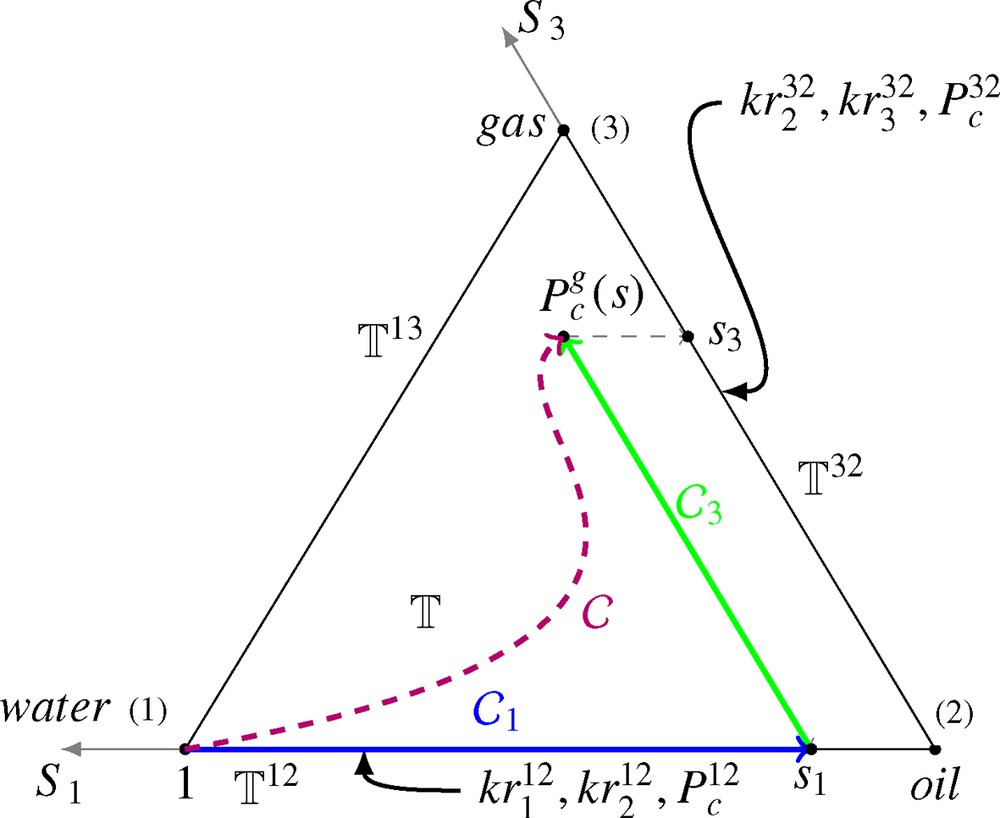Fig. 1Illustration of the ternary diagram T and the location of the required two-phase data used in approach A.Schéma du diagramme ternaire T et des données diphasiques requises dans l’approche A.

This TD equivalent condition yields two simple linear equations, which can be solved on the ternary diagram using (7) and the three-phase oil fractional flow f2. Therefore, we are able to build the global capillary function $Pcg$ (Chavent, 2009; di Chiara Roupert et al., 2010). The global pressure varies between water pressure and gas pressure, then $Pcg(1,0,P)=0$ at the water vertex. The TD condition (see (5)) can be re-written for all s in T in an equivalent form:

 $∂Pcg∂s1(s,p)=f1(s,p−Pcg)dPc12ds1(s1),∂Pcg∂s3(s,p)=f3(s,p−Pcg)dPc32ds3(s3).$ (9)

Therefore, the global capillary function $Pcg$ must satisfy a differential equation along any given smooth curve C in T (see Fig. 1). From the previous step, the TD fractional flow functions allow the construction of the global capillary function $Pcg$ using the two two-phase capillary pressure gradients and using a convenient path (see Fig. 1) along the water-oil side (curve C1) and gas-oil side (curve C3):

 $Pcg(s,p)=∫1s1f1(σ,0,p)dPc12ds1(σ)dσ+∫0s3f3(s1,σ,p)dPc32ds3(σ)dσ.$ (10)

Following Chavent and Salzano (1985) and Chavent and Jaffré (1986), the next step determines d on the third side, that is, the water-gas side of T. The global mobility of the water-gas side d13must be determined carefully. Otherwise, corresponding relative permeabilities $kr113$ for water and $kr313$ for gas computed from (7) may range out of the interval; they also may decrease in directions in which one would expect them to increase. Therefore, a constrained optimization problem is built up on the relative permeabilities using previous TD fractional flows for water and for gas to obtain the corresponding TD relative permeabilities. Once the ternary diagram T is closed using the initial values on the water-oil boundary T12, the gas-oil boundary T32 and optimised TD data on T13, the global mobility is assessed for T. Similarly, two additional constrained optimization problems are solved to compute TD three-phase permeabilities and, therefore, global mobility; see equation (7). At the end of optimization procedure, the corresponding TD three-phase permeabilities shall have a Laplacian value that is not too large but ranges between 0 to 1 and strictly increases towards the phase-related vertex of T (Jégou, 1997; Chavent and Salzano, 1985; Chavent and Jaffré, 1986). Bound constraints and regularity behaviour are set for the objective functions of the TD data using a Quasi-Newton algorithm (Byrd et al., 1994). The resulting problem is weakly parameterized, a damped or trust region Gauss-Newton method like Levenbeg-Marquardt, or a “Dog Leg” method could also be implemented to solve sequentially the optimization issue. Details of the construction of objective functions, their gradients and linear-bound constraints are given in di Chiara Roupert (2009).

At the end of the procedure, global mobility d(s,p), fractional flows fj(s,p) and global capillary function $Pcg(s,p)$ are known and, thus, are used to solve the global pressure equation (4), the global volumetric flow rate (6) and the saturation equations (8).

### 3.2 New TD interpolation by finite elements (approach B)

In the exact global pressure formulation, d, f1, f2, f3, and $ρ¯$ are functions expressed by the oil pressure level p2; see equation (4). Initial two-phase flow data are set on water-oil side T12, gas-oil side T32 and water-gas side T13, gas-oil side and water-gas side (see Fig. 2). The reader may refer to di Chiara Roupert et al. (2010) for details of the algorithm we use here.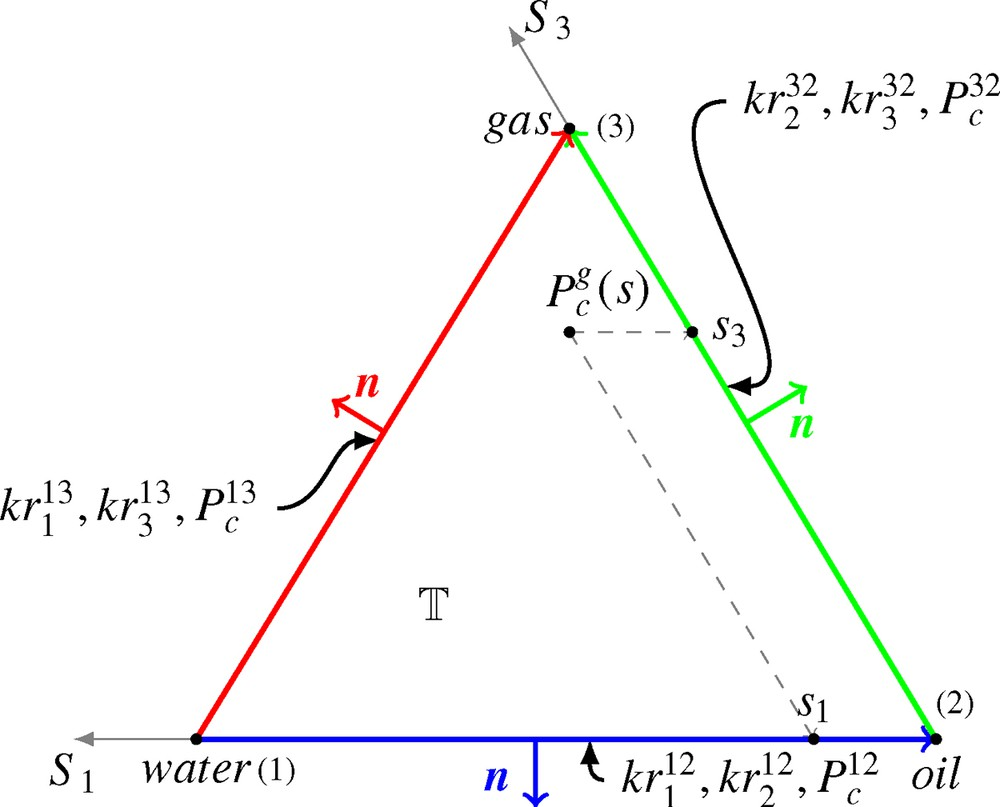Fig. 2Illustration of the ternary diagram T and the location of the required two-phase data used in approach B.Schéma du diagramme ternaire T et des données diphasiques requises dans l’approche B.

We solve first the initial value problems on the three two-phase sides of the ternary diagram T. The three ODEs are built up from tangential derivatives of $Pcg$ over the three sides of the ternary diagram. Their expressions are deduced from relations (9) and solved with an adaptative predictor-corrector scheme (di Chiara Roupert, 2009; Quarteroni et al., 2007). Therefore, the global capillary function is calculated on the three two-phase boundaries.

To satisfy the TD condition on the boundaries, the global capillary function must be slightly modified on the gas-oil side to provide an exact matching value at the gas vertex (Chavent, 2009; di Chiara Roupert, 2009; di Chiara Roupert et al., 2010).

The three two-phase global mobilities d on boundaries are then computed (see Eq. (7)) with P replaced by $P2=P−Pcg(s,p)$. The global mobility is then obtained from previous Dirichlet boundary condition by solving a harmonic equation on T by means of C0 piecewise linear finite elements.

In the last step, the global capillary pressure is extended to the entire ternary diagram T. It is necessary to complete the Dirichlet conditions known from ODE calculations with Neumann conditions along the same boundaries (see Fig. 2). Thus the problem amounts to enquire on each node the function $Pcg$ and its normal derivative on each mid-side node belonging to the border (Chavent, 2009; di Chiara Roupert et al., 2010). Normal derivatives are computed as linear combinations of derivatives $∂Pcg/∂s1$ and $∂Pcg/∂s3$ from (9). A bi-harmonic equation over is needed to provide a continuous global capillary pressure field. It is solved using a C1 Hsieh-Clough-Tocher finite element method (Bernadou and Hassan, 1980; Clough and Tocher, 1965), which ensures that the fractional flows derived from $Pcg$ are continuous over the entire ternary diagram. At the end of the C1 finite element interpolation, $Pcg$, $∂Pcg/∂s1$ and $∂Pcg/∂s3$ are provided. For each fluid phase, the corresponding fractional flow is then derived for j = 1,3 using:

 $fj(s,p)=(∂Pcg/∂sj(s,p))/(dPcj2/dsj(sj)),$ (11)
with oil fractional flow given by the closure relation f2 = 1 − f1 − f3. Similarly to approach A, global mobility d(s,p), fractional flows fj (s,p) and global capillary function $Pcg(s,p)$ are known and, thus, used to solve the global pressure equation and saturation equations.

## 4 A comparison of numerically derived three-phase TD data

In this section, we compare the water fractional flow f1, the global mobility d and the global capillary pressure $Pcg$ obtained from approach A and approach B for an incompressible three-phase flow (ω=1). Input data were chosen for a typical fine sand; we considered the van Genuchten model function (Van Genuchten, 1980) coupled with Parker's normalisation method (Parker et al., 1987) to express the capillary pressure-saturation relationship in the two-phase systems for water-oil $Pc12$ and gas-oil $Pc32$. Specifically, we used both the Van Genuchten parameters (α and n) in the two-phase gas-water system and the corresponding surface tensions of water-oil and gas-oil. The perfect gas law is used at a temperature of T = 293 K. All parameters used in the case study are given in Table 1. Here, the oil phase is made of trichloroethylene (TCE). In terms of wettability, water is the wetting phase in the water-oil system and oil is the wetting phase in the gas-oil system. Consequently, water is the wetting phase in the water-gas system. The resulting TD water fractional flows are shown in Figs. 3 and 4. Differences occur on the water-gas side T13 because optimization does not take into consideration any relative permeabilities or initial capillary pressures. In Fig. 4, numerical results obtained from the bi-harmonic problem are given; $Pcg$, $∂Pcg/∂s1$ and $∂Pcg/∂s3$ and are computed using the three two-phase data given on the sides as Dirichlet and Neuman boundary conditions.

Table 1

Données initiales utilisées pour les trois systèmes diphasiques.

 Symbol Value Description Unit α 0.0001 α Van Genuchten parameter, fine sand [Pa−1] m 0.93 m Van Genuchten parameter, fine sand [–] μ 1 1.2 Water dynamic viscosity [×10−3Pa.s] μ 2 0.55 Oil dynamic viscosity [×10−3Pa.s] μ 3 0.015 Gas dynamic viscosity [×10−3Pa.s] σ13/σ32 2.5 Gas-oil dimensionless surface tension [–] σ13/σ12 2.1 Water-oil dimensionless surface tension [–] B j 1 Volume factor for j = 1, 2, 3 [–] p 1 Global pressure level [bar]Fig. 3Water fractional flow f1 obtained with approach A.Flux fractionnaire en eau f1 obtenu suivant l’approche A.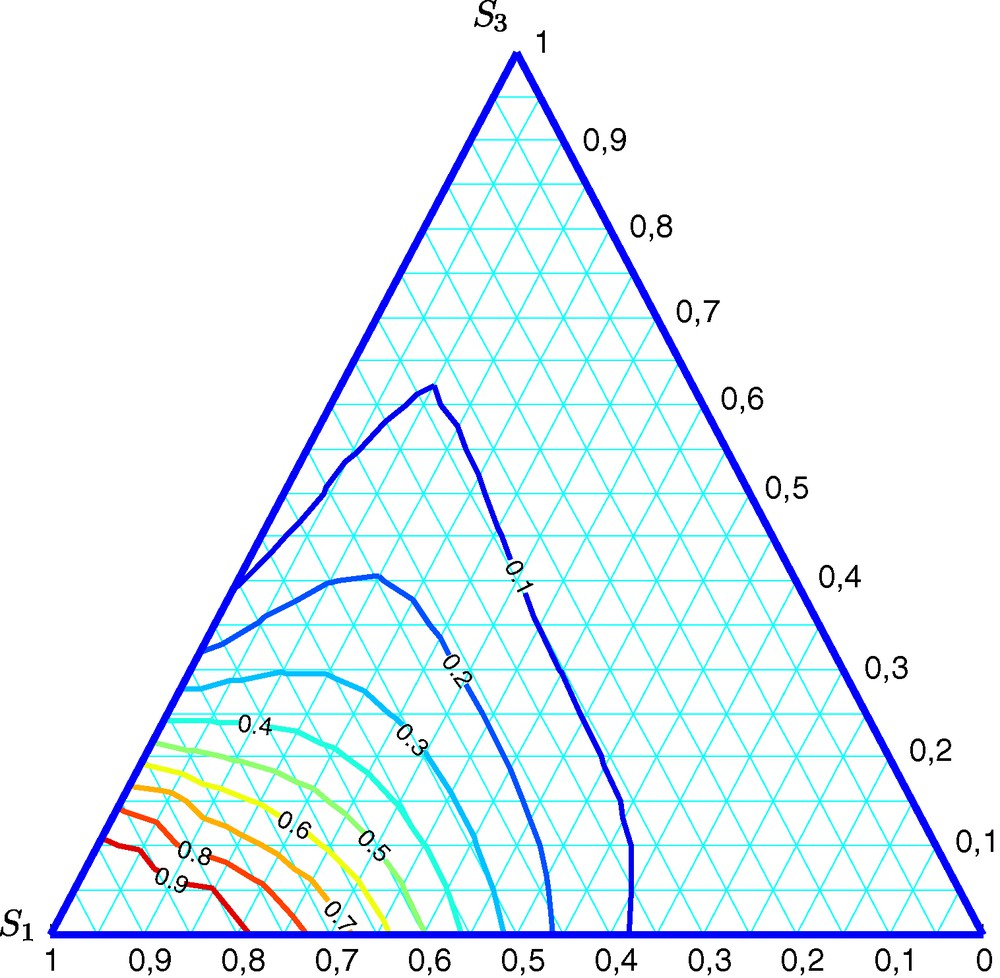Fig. 4Water fractional flow f1 obtained with approach B.Flux fractionnaire en eau f1 obtenu suivant l’approche B.

TD fractional flows computed from (11) exactly match the given initial models of two-phase capillary pressures and relative permeabilities (see Fig. 2). To compare both approaches, we calculated relative differences at the point of the ternary diagram at which s1 = 45% and s3 = 40%. At this point, the TD fractional flow obtained with approach A is about 25% higher than the one evaluated with approach B. In this case, the water volumetric flow vector (8) is higher in approach A than B. Figs. 5–7 show the global capillary function obtained from the optimization approach and the C1 finite element approach, respectively. Resulting values vary in the same range. One can see that the initial value $Pcg(1,0,p)=0$ is satisfied at the maximum water saturation. Both functions grow very rapidely near the gas vertex due to two-phase capillary gradients. Each computed function $Pcg=P−P2$ shows a regular behaviour near the maximum oil saturation. It shows that for both methods, global pressure is a more appropriate unknown than oil pressure, as p2 − pj is singular near the jth vertex. Figs. 8 and 9 show global mobility as obtained from the optimization approach and the C0 finite element approach. In Fig. 8, the resulting TD global mobility is expected to be regular and smooth over T. Due to the water-gas optimised value found on T13, the TD global mobility field is quite different in the water-gas region compared to the gas-oil region. To discuss differences between approach A and B, relative differences are calculated at s1 = 25%, s1 = 65%. At this point, the TD global mobility as predicted by approach B is about 50% higher than the value calculated by approach A. Hence, in that particular case, the TD global mobility as obtained by the new TD interpolation scheme (approach B) allows the global volumetric flow vector (6) to achieve higher values than that provided by approach A. In the vicinity of the oil vertex, TD global mobility is the same as that obtained using Stone's model, which is not really surprising as it has been chosen as the oil fractional flow model f2 in approach A (see Section 3.1). Here, mobilities which are built in approach A are based on an approximated formulation of the global pressure while results of approach B are obtained with the exact formulation: the volume factors Bj are measured at pressure level pj and not at the global pressure level p. If the two approaches are both TD compatible, the global mobility obtained in approach A (see Figs. 8 and 9) will tend to underestimate the total flux for s2 < 40% compared to approach B. This situation represents the majority of cases encountered in real pollution situations with a plume at residual oil saturation (s2 <<< 40%). Furthermore, we present specific results for $Pcg$ data on T13 (see Fig. 7, left). In the case of approach B, the resulting water-gas capillary pressure curve exactly fits the Van Genuchten model, whereas at low water saturations, the capillary pressure values of approach A are several times higher than the physical data. This difference is a consequence of the sharp contrast of the global mobility towards the gas vertex. This difference is due to the TD relative permeabilities optimized on the water-gas side (approach A). In approach A, the total flow will thus be underestimated for three-phase flows where the gas saturation is high.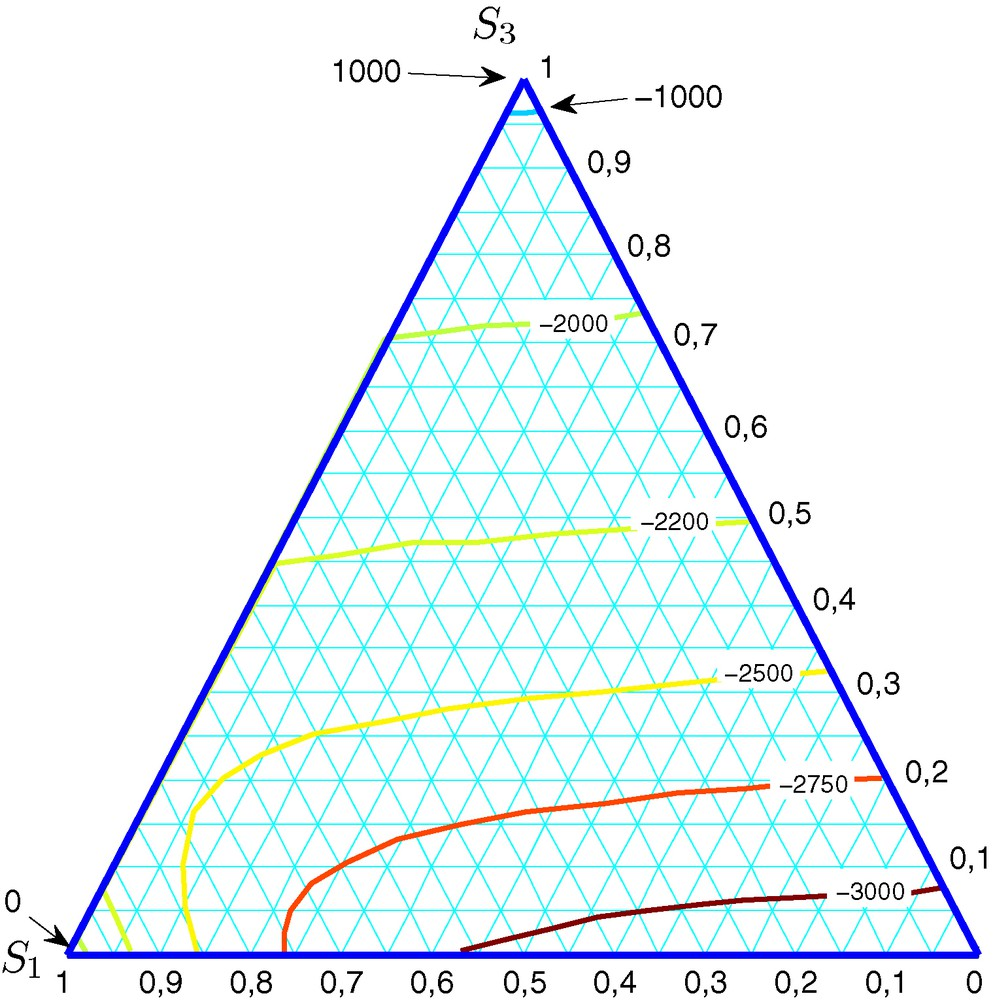Fig. 5Global capillary pressure Pcg obtained with approach A.Pression capillaire globale Pcg obtenue avec l’approche A.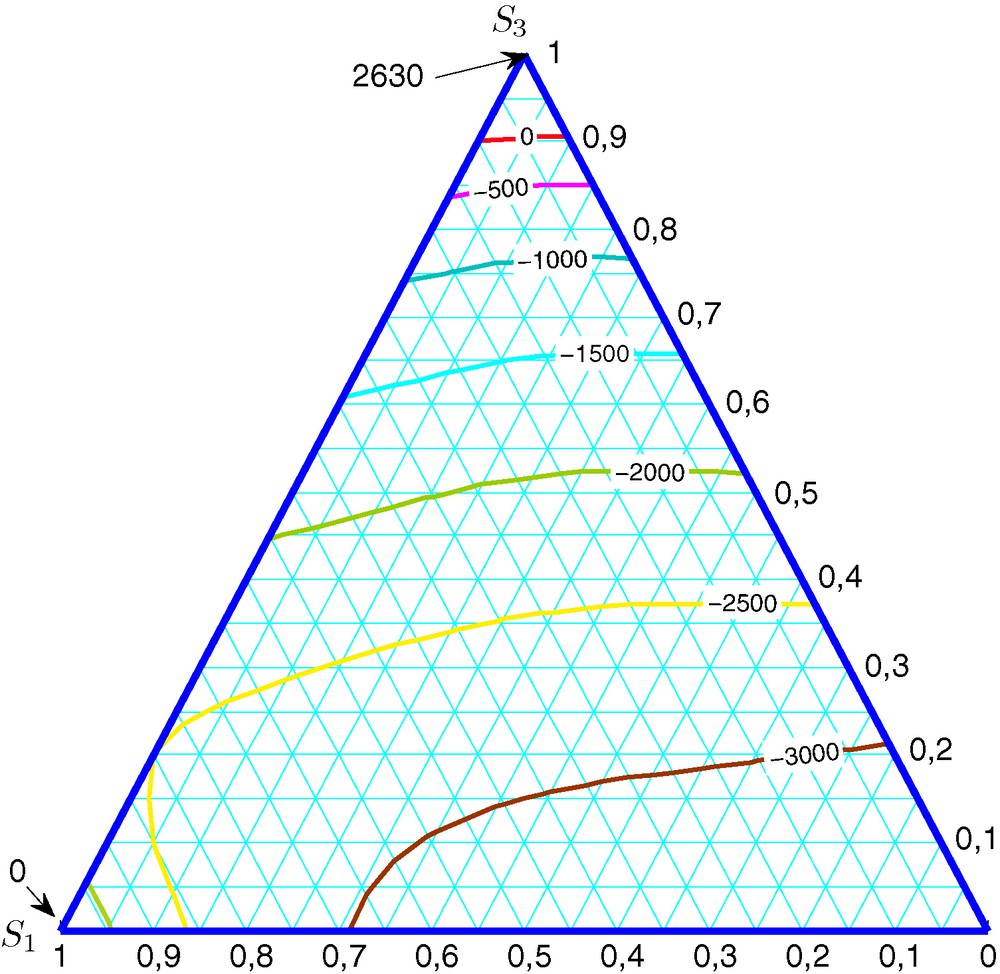Fig. 6Global capillary pressure Pcg obtained with approach B.Pression capillaire globale Pcg obtenue avec l’approche B.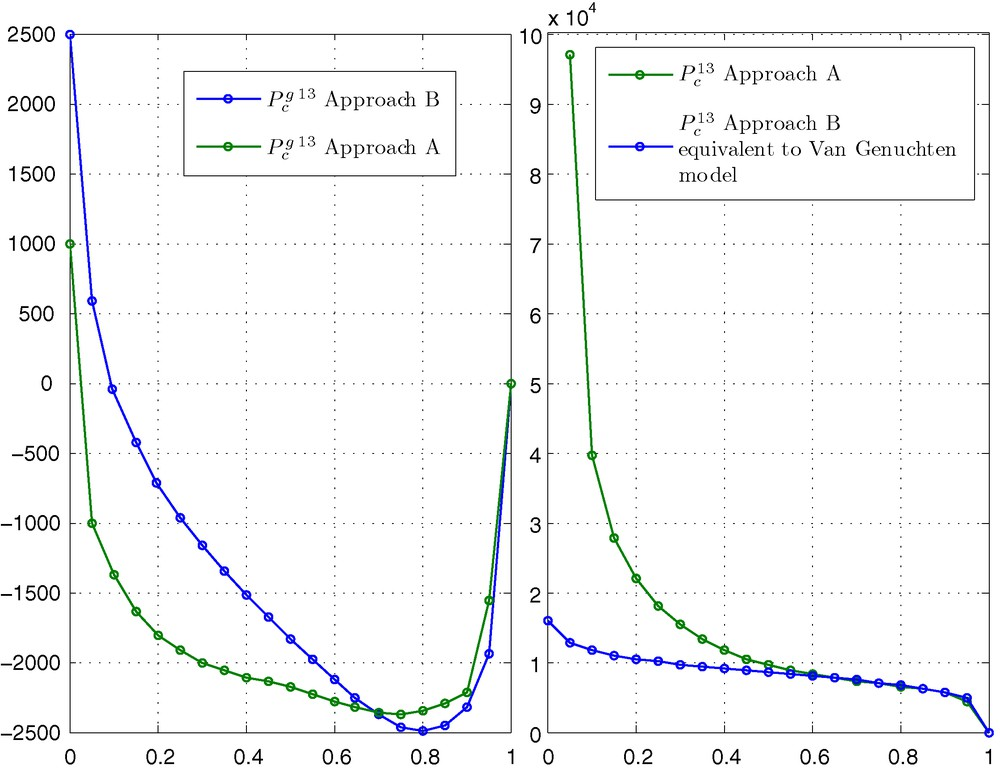Fig. 7Global capillary pressure Pcg13 (left) and capillary pressure Pc13 (right) calculated on the water-gas side.Pression capillaire globale Pcg13 (gauche) et pression capillaire Pc13 (droite) calculées sur le côté eau-gaz.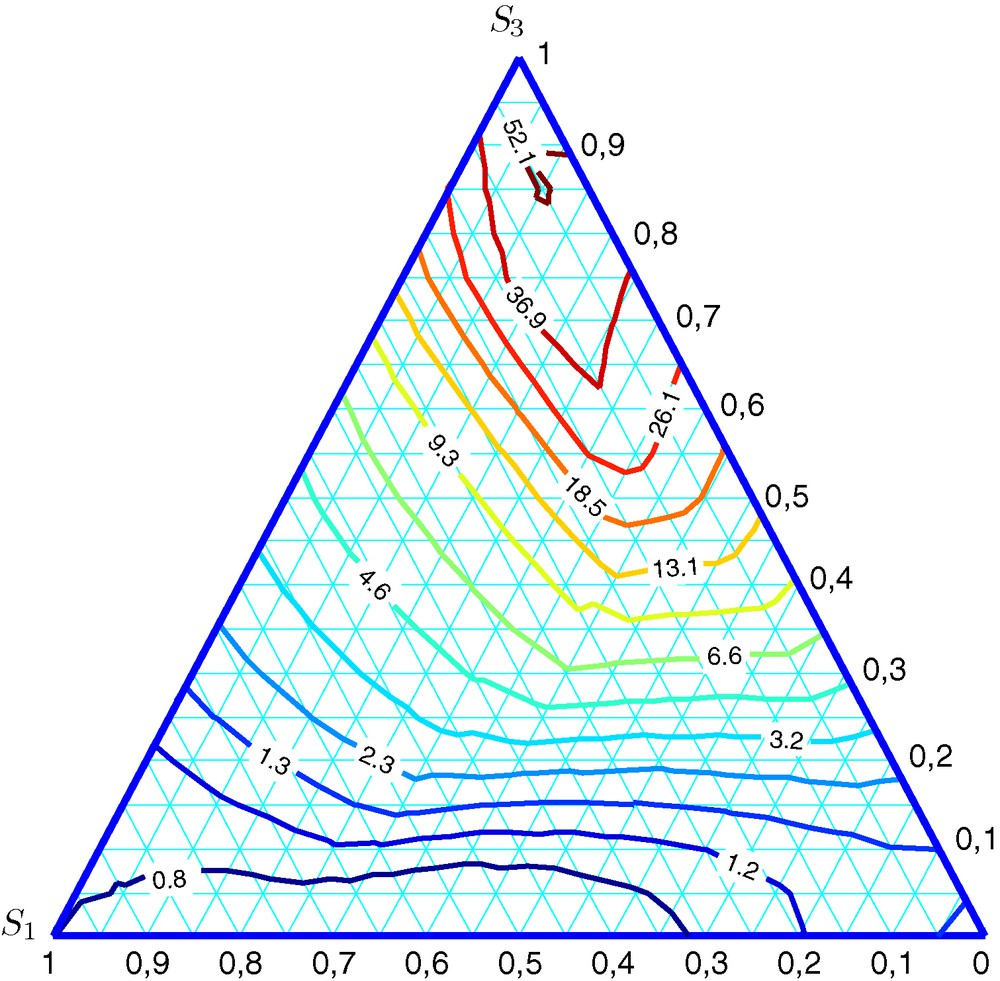Fig. 8Global mobility d obtained with approach A.Mobilité globale d obtenue suivant l’approche A.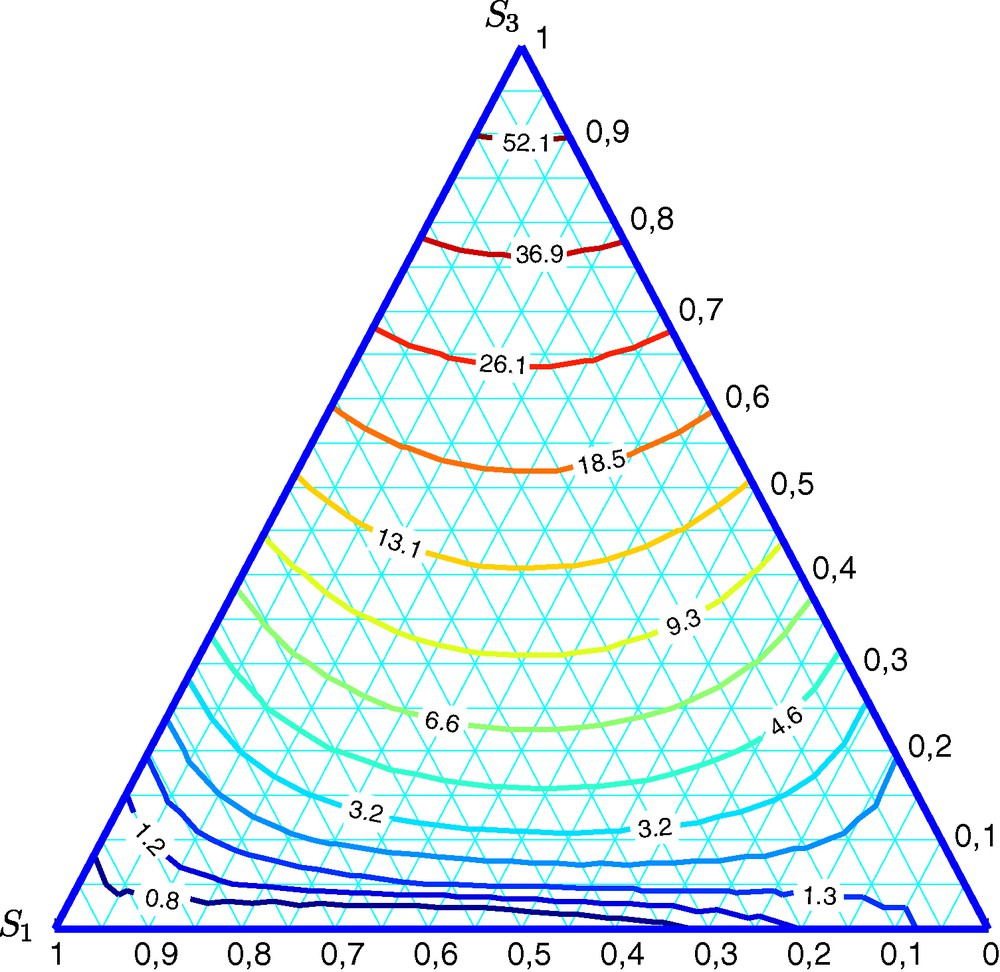Fig. 9Global mobility d obtained with approach B.Mobilité globale d obtenue suivant l’approche B.

## 5 Conclusion

In this article, we analysed two three-phase parameterisation approaches to assess the TD secondary variables required for efficient multiphase flow simulators using a global pressure formulation. Usually, experimental three-phase data are rarely investigated inside the three-phase water-oil-gas diagram (Bano et al., 2009; Oak et al., 1990). From a practical point of view, these two TD interpolations have given similar CPU times within the order of a second. They represent robust and innovative approaches for efficiently modelling three-phase flow equations. Both methods satisfy the TD condition and allow the three-phase compressible flow equation to be rewritten in a more suitable fractional form; see equations (6) and (8). However, by construction, the resulting two-phase water-gas data, such as the capillary pressure curve, differ between the original and the new TD interpolation. When flow takes place only in a two-phase water-gas system, TD three-phase data obtained by the optimization (approach A) might be significantly different from that derived using the finite element interpolation (approach B). It also may differ from the data from methods using classical capillary curves, such as the Van Genuchten model, as is often employed in the field of environmental sciences. As shown in Fig. 7, the finite element approach seems to be more suitable for explicitly taking into account two-phase capillary pressure and relative permeability data in a water-gas system. Furthermore, optimization parameters may be difficult to select. They depend on the governing parameters of the simulated cases (eg. viscosities, pressure levels, etc.) and more specifically initial values for the two relative permeability models used on the water-gas side optimization. Moreover, numerical tests (see approach A) showed that the global mobility field heavily depends on dynamic viscosity of the gas phase. Therefore, a finite element interpolation approach combined with a flow simulator seems to be better suited to generate three-phase flow variables than the optimization approach. In other words, the finite element approach appears to be a robust and efficient solution for three-phase flow simulation. At the reservoir scale, we can expect these differences to have little impact on the flow behaviour, as it is knwon that the shock saturation mainly dominates two phase flows. Note that in the TD approach, Chavent and Jaffré (1986) showed that the hyperbolicity condition is not fully verified particularly for large oil saturation values in the first Stone model. This condition should be satisfied by any three-phase data set if one wants small capillary pressure problems to be well posed for any values of volume factors. Besides, Stone's models are not TD compatible and therefore do not allow reformulation of the flow in a simplified way (see (6). Further research will be focussed on more systematic numerical flow comparisons with other three-phase correlations.

## Acknowledgements

The authors would like to thank Professor Frédéric Delay from the University of Poitiers and the four other anonymous referees for their valuable comments and suggestions which helped improve the manuscipt significantly. Financial support for this research from the Programme National de Recherche en Hydrologie (INSU-CNRS) and the Agence De l’Environnement et de la Maîtrise de l’Energie (ADEME) is gratefully acknowledged. We would also like to thank ADEME and Burgéap SA for financing the PhD of R. di Chiara Roupert.

References

[Amaziane and Jurak, 2008] B. Amaziane; M. Jurak A new formulation of immiscible compressible two-phase flow in porous media, C. R. Mecanique, Volume 336 (2008), pp. 600-605

[Antoncev and Monahov, 1978] S.N. Antoncev; V.N. Monahov Three-dimensional problems of time-dependant two-phase filtration in non-homogeneous anisotropic porous media, Soviet Math. Doklady, Volume 19 (1978), pp. 1354-1358

[Baker, 1988] Baker, L.E., 1988. Three-Phase Relative Permeability Correlations, paper SPE 17369 presented at the 1988 SPE/DOE Enhanced Oil Recovery Symposium, Tulsa, Oklahoma.

[Bano et al., 2009] M. Bano; O. Loeffler; J.-F. Girard Ground penetrating radar imaging and time-domain modelling of the infiltration of diesel fuel in a sandbox, C. R. Geoscience, Volume 341 (2009), pp. 846-858

[Bear, 1972] J. Bear Dynamics of Fluids in Porous Media, Elsevier, New York, 1972

[Benremita and Schäfer, 2003] H. Benremita; G. Schäfer Transfert du trichloréthylène en milieu poreux à partir d’un panache de vapeurs, C. R. Mecanique, Volume 331 (2003), pp. 835-842

[Bernadou and Hassan, 1980] M. Bernadou; K. Hassan Basis functions for general Hsieh-Clough-Tocher triangles complete or reduced, Inria Research Report RR-5 (1980)

[Binning and Celia, 1999] P. Binning; M.A. Celia Practical implementation of the fractional flow approach to multi-phase flow simulation, Adv. Water Resour., Volume 22 (1999) no. 5, pp. 461-478

[Bohy et al., 2004] M. Bohy; G. Schäfer; O. Razakarisoa Caractérisation de zones sources de DNAPL à l’aide de traceurs bisolubles: mise en évidence d’une cinétique de partage, C. R. Geoscience, Volume 336 (2004), pp. 799-806

[Bohy et al., 2006] M. Bohy; L. Dridi; G. Schäfer; O. Razakarisoa Transport of a mixture of chlorinated solvent vapors in the vadose zone of a sandy aquifer, Vadose Zone Journal, Volume 5 (2006), pp. 539-553

[Byrd et al., 1994] R. Byrd; P. Lu; J. Nocedal; C. Zhu A limited memory algorithm for bound constrained optimization, Computer Science Department, University of Colorado at Boulder NAM-08 (1994)

[Chavent, 2009] G. Chavent A Fully Equivalent Global Pressure Formulation for Three-Phase Compressible Flow, Applicable Analysis, Volume 88 (2009) no. 10, pp. 1527-1541

[Chavent and Salzano, 1985] G. Chavent; G. Salzano Un algorithme pour la détermination de perméabilités relatives triphasiques satisfaisant une condition de différentielle totale, Inria Research Report RR-0355 (1985)

[Chavent and Jaffré, 1986] G. Chavent; J. Jaffré Mathematicals Models and Finite Elements fo Reservoir Simulation, North-Holland, Amsterdam (1986)

[Chavent et al., 2008] Chavent G, di Chiara Roupert R, Schäfer G, 2008 A Fully Equivalent Global Pressure Formulation for Three-Phase Compressible Flows, Conference Scaling’Up 08, Dubrovnik.

[Chen, 2005] Z. Chen Finite Element Methods and Their Applications, Scientific Computation, Springer, Berlin, 2005

[Chen and Ewing, 1997] Z. Chen; R. Ewing Comparison of Various Formulations of Three-Phase Flows in Porous Media, Journal of Computational Physics, Volume 132 (1997), pp. 362-373

[Clough and Tocher, 1965] R.W. Clough; J.L. Tocher Finite element stiffness matrices for analysis of plate bending, Proceedings of the Conference on Matrix Methods in Structural Mechanics, Wright Patterson A.F.B, Ohio, USA, 1965

[Cohen and Mercer, 1993] R.M. Cohen; J.W. Mercer DNAPL site evaluation (C.K. Smoley, ed.), CRC Press, Florida, USA, 1993

[di Chiara Roupert, 2009] R. di Chiara Roupert Développement d’un code de calcul multiphasique multiconstituants, PhD thesis, Université de Strasbourg, France (2009)

[di Chiara Roupert et al., 2010] R. di Chiara Roupert; G. Chavent; G. Schäfer Three-phase compressible flow in porous media: total Differential Compatible interpolation of relative permeabilities, Journal of Computational Physics, Volume 229 (2010), pp. 4762-4780 | DOI

[Dridi et al., 2009] L. Dridi; I. Pollet; O. Razakarisoa; G. Schäfer Characterisation of a DNAPL source zone in a porous aquifer using the Partitioning Interwell Tracer Test and an inverse modelling approach, Journal of Contaminant Hydrology (2009) | DOI

[Helmig, 1997] R. Helmig Multiphase flow and transport processes in the subsurface. A contribution to the modeling of hydrosystems. Springer (Environmental Engineering), 1997

[Jégou, 1997] S. Jégou Estimation des perméabilités relatives dans des expériences de déplacements triphasiques en milieu poreux, Thése, Université Paris IX Dauphine, France, 1997

[Nayagum et al., 2001] D. Nayagum; G. Schäfer; R. Mose Approximation par les éléments finis mixtes d’une équation de diffusion non linéaire modélisant un écoulement diphasique en milieu poreux, C. R. Acad. Sci Paris, Volume 239 (2001), pp. 87-90

[Nayagum et al., 2004] D. Nayagum; G. Schäfer; R. Mose Modeling two-phase incompressible flow in porous media using mixed hybrid and discontinuous finite elements, Computational Geosciences, Volume 8 (2004), pp. 49-73

[Nex et al., 2006] F. Nex; G. Schäfer; J.M. Côme; T.M. Vogel Une approche innovante pour modéliser la biodégradation des composés organo-chlorés volatils en aquifères poreux, C. R. Geoscience, Volume 338 (2006), pp. 287-306

[Oak et al., 1990] M.J. Oak; L.E. Baker; D.C. Thomas Three-Phase Relative Permeability of Berea Sandstone, Journal of Petroleum Technology Trans., AIME, Volume 1054 (1990), p. 289

[Parker et al., 1987] J.C. Parker; R.J. Lenhard; T. Kuppusami Parametric model for constitutive properties governing multiphase flow in porous media, Water Resour. Res., Volume 23 (1987), pp. 618-624

[Quarteroni et al., 2007] A. Quarteroni; R. Sacco; F. Saleri Méthodes Numériques – Algorithme, analyse et applications, Springer, Italia, 2007

[Sinke and Le Hecho, 1999] A. Sinke; I. Le Hecho Monitored natural attenuation: review of existing guidelines and protocols, TNO-Nicole report, Apeldoorn, The Netherlands, 1999

[Stone, 1970] H.L. Stone Probability model for estimating three-phase relative permeabilitiy, Journal of Petroleum Technology, Volume 22 (1970), pp. 214-218

[Stone, 1973] H.L. Stone Estimation of Three-Phase Relative Permeability and Residual Oil Data, J. Cdn. Pet. Tech., Volume 12 (1973)

[Van Genuchten, 1980] M.T. Van Genuchten A closed-form equation for predicting the hydraulic conductivity of unsaturated soils, Soil Sci. Soc. Am. J., Volume 44 (1980), pp. 892-989

Articles of potential interest

A new formulation of immiscible compressible two-phase flow in porous media